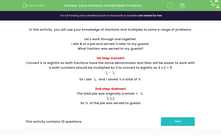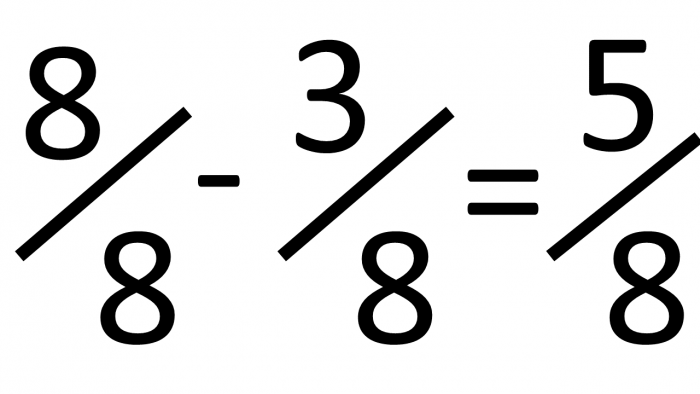# Find Lowest Common Multiples to Solve Fraction Problems

In this worksheet, students will be asked to solve a range of fraction problems using lowest common multiples.Key stage:  KS 2

Curriculum topic:   Ratio and Proportion

Curriculum subtopic:   Solve Fraction/Multiples Problems

Difficulty level:#### Worksheet Overview

In this activity, we will use our knowledge of fractions and multiples to solve a range of problems.

Let's work through one together.

I ate ¼ of a delicious apple pie and saved  to eat later.

I gave the rest of the pie to some friends who dropped in.

What fraction was served to my friends?1st step: Convert

Convert ¼ to eighths so that both fractions have the same denominator and then will be easier to work with.

4 x 2 = 8 so we need to multiply both the nominator and the denominator by 2 to change ¼ into eighths.So I ateand I saved          A total of ⅜

2nd step: Subtract

The total pie was originally a whole =So ⅝  of the apple pie was served to my friends.

Let's move on to the questions now.

### What is EdPlace?

We're your National Curriculum aligned online education content provider helping each child succeed in English, maths and science from year 1 to GCSE. With an EdPlace account you’ll be able to track and measure progress, helping each child achieve their best. We build confidence and attainment by personalising each child’s learning at a level that suits them.

Get started